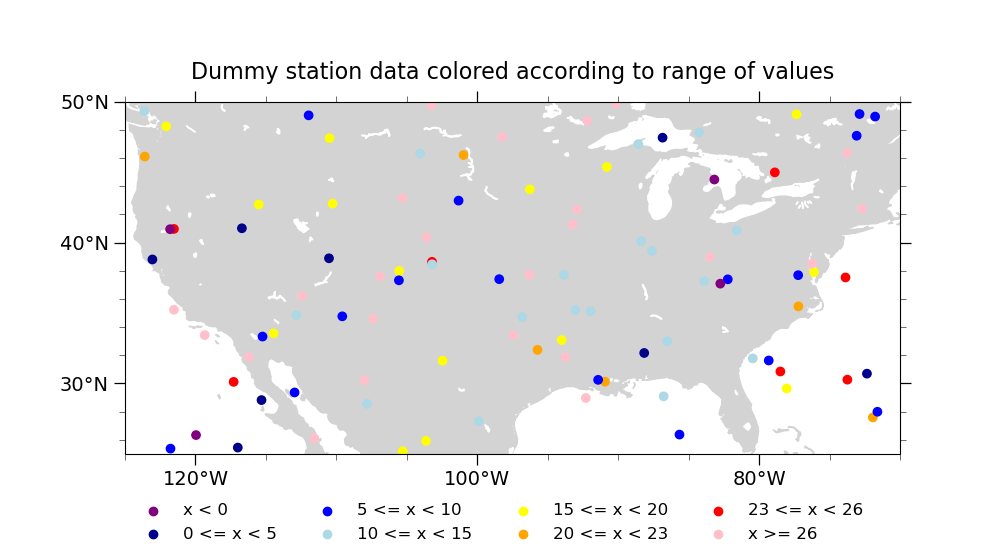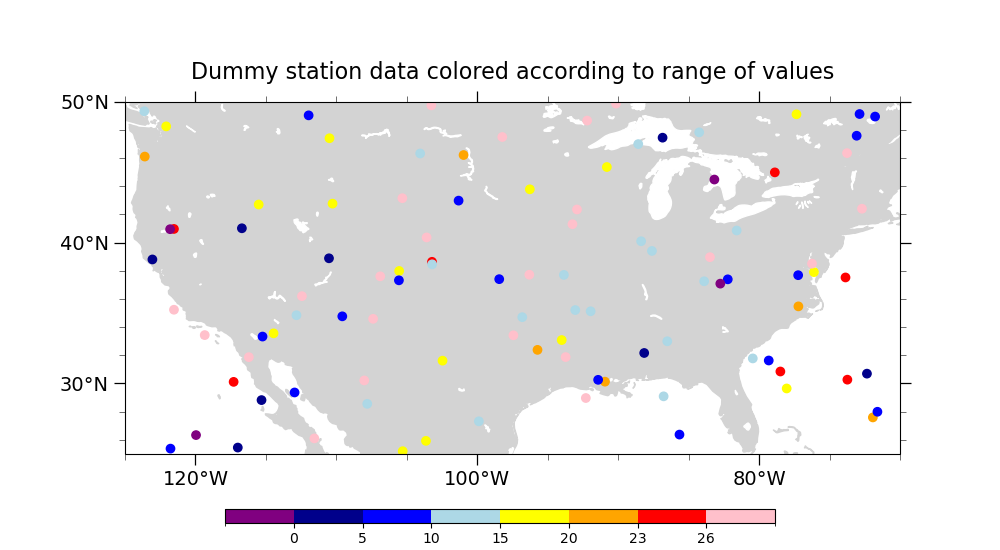# NCL_station_2.py#

This script illustrates the following concepts:
• Drawing markers on a map indicating the locations of station data

• Generating dummy data using “random_uniform”

• Drawing markers of different sizes and colors on a map

• Drawing a custom legend outside of a map plot

• Attaching a custom colorbar to a plot

See following URLs to see the reproduced NCL plot & script:

Import packages:

```import numpy as np
import matplotlib as mpl
from matplotlib import pyplot as plt
import cartopy
import cartopy.crs as ccrs

import geocat.viz as gv
```

## Generate random data:#

```# Set up random values
npts = 100
np.random.seed(20200127)

# Lat between 25 N and 50 N, lon between 125 W and 70 W
lat = np.random.uniform(25, 50, npts)
lon = np.random.uniform(235, 290, npts) - 360

dummy_data = np.random.uniform(-1.2, 35, npts)
```

## Define colormap for plotting:#

```# Set up colormap:
# Need to define boundaries for each color map as well as colors for each bin

# Note that len(colors) = len(bin_bounds) + 1
# color => dummy_data < bin_bounds
# color[-1] => dummy_data => bin_bounds[-1]
# color[j] => bin_bounds[j-1] <= dummy_data < bin_bounds[j]

bin_bounds = [0., 5., 10., 15., 20., 23., 26.]
colors = [
'purple', 'darkblue', 'blue', 'lightblue', 'yellow', 'orange', 'red', 'pink'
]

nbins = len(colors)  # One bin for each color

# Define colormap for plotting based on these colors
cmap = mpl.colors.ListedColormap(colors)
```

## Utility Function: Make Shared Plot:#

```# Define a utility function to create the basic contour plot that will be used twice to create two slightly
# different plots, both of which rely on same base figure
def make_shared_plot(title):

# Generate figure (set its size (width, height) in inches) and axes using Cartopy projection
plt.figure(figsize=(10, 5.5))
ax = plt.axes(projection=ccrs.PlateCarree())

# Use geocat.viz.util convenience function to add minor and major tick lines
x_minor_per_major=4,
y_minor_per_major=5,
labelsize=14)

# Use geocat.viz.util convenience function to make plots look like NCL plots by using latitude, longitude tick labels

# Use geocat.viz.util convenience function to set axes limits & tick values without calling several matplotlib functions
gv.set_axes_limits_and_ticks(ax,
xlim=(-125, -70),
ylim=(25, 50),
xticks=range(-120, -75, 20),
yticks=range(30, 51, 10))

edgecolor='lightgray',
facecolor='lightgray',
zorder=0)
edgecolor='white',
facecolor='white',
zorder=0)

# Scatter-plot the location data on the map
scatter = plt.scatter(lon, lat, c=dummy_data, cmap=cmap, zorder=1)

plt.title(title, fontsize=16, y=1.04)

return scatter, ax
```

## Plot 1 (with a legend outside, i.e. station_2_1.png)#

```# Draw the base plot
scatter1, ax = make_shared_plot(
"Dummy station data colored according to range of values")

# Add a legend to the bottom outside of the plot
# Given how we generated the plot, adding a legend is a little kludgy. Basically, we draw a second plot where no data
# is in frame but the legend for that plot is drawn where we want it
lax = plt.axes((0, 0, 1, 0.1), frameon=False)

# Plotting window is [0,1] x [0,1]
plt.xlim(0, 1)
plt.ylim(0, 1)
plt.axis('off')

for n, color in enumerate(colors):
if n == 0:
label = f'x < {bin_bounds:.0f}'
elif n == nbins - 1:
label = f'x >= {bin_bounds[-1]:.0f}'
else:
label = f'{bin_bounds[n-1]:.0f} <= x < {bin_bounds[n]:.0f}'

# Plotting data at (-10, -10) which is not in the plotting window
scatter = plt.scatter(-10, -10, color=color, label=label)

# The legend for this second plot is what we are actually interested in
# We want large font, no frame around the legend, and 4 columns of labels
lax.legend(loc='center', fontsize='large', frameon=False, ncol=4)

plt.show()
```## Plot 2 (with a colorbar, i.e. station_2_2.png)#

```# Draw the base plot
scatter2 = make_shared_plot(
"Dummy station data colored according to range of values")

cax = plt.axes((0.225, 0.05, 0.55, 0.025))
norm = mpl.colors.BoundaryNorm([-1.2] + bin_bounds + , len(colors))
mpl.colorbar.ColorbarBase(cax,
cmap=cmap,
orientation='horizontal',
norm=norm,
boundaries=[-1.2] + bin_bounds + ,
ticks=bin_bounds)

# Show the plot
plt.show()
```Total running time of the script: ( 0 minutes 0.412 seconds)

Gallery generated by Sphinx-Gallery